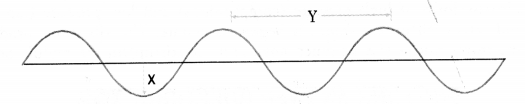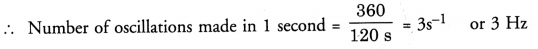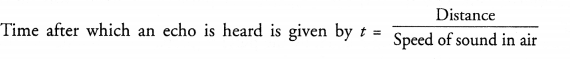## HOTS Questions for Class 9 Science Chapter 12 Sound

These Solutions are part of HOTS Questions for Class 9 Science. Here we have given HOTS Questions for Class 9 Science Chapter 12 Sound

Question 1.
Sound of explosions taking place on other planets are not heard by a person on the earth. Explain, why ?
(CBSE 2011)
Sound needs material medium for its propagation from one place to another place. In other words, sound cannot travel through vacuum. Since there is a region in between the planets and the earth, where there is a vacuum, so the sound of explosions taking place on other planets cannot pass through this vaccum. Hence cannot reach the earth.

More Resources

Question 2.
Two astronauts on the surface of the moon cannot talk to each other. Explain, why ?
Since there is no atmosphere on the surface of the moon (i.e. no medium for the propagation of sound), so the sound cannot travel from one astronaut to another astronaut on the surface of the moon.

Question 3.
A loud sound can be heard at a large distance but a feeble or soft sound cannot be heard at a large distance. Explain, why ?
Sound is a form of energy which is transferred from one place to another place. As sound energy is directly proportional to the square of the amplitude of a vibrating body, so loud sound has large energy, whereas soft sound has small energy. As the sound travels through a medium, sound with small energy is absorbed after travelling a small distance in the medium but sound with large energy will be absorbed after travelling a large distance in the medium. Therefore, loud sound can be heard at a large distance but feeble sound cannot be heard at a large distance.

Question 4.
A sound wave travelling in a medium is represented as shown in figure,

1. Which letter represents the amplitude of the sound wave ?
2. Which letter represents the wavelength of the wave ?
3. What is the frequency of the source of sound if the vibrating source of sound makes 360 oscillations in 2 minutes ?1. Letter X represents the amplitude of the sound wave.
2. Letter Y represents the wavelength of the sound wave.
3. Number of oscillations made in 2 minutes (120 s) = 360Hence, frequency of the source of sound = 3 Hz

Question 5.
Represent the following sound waves,
(i) Waves having same amplitude but different frequencies
(ii) Waves having same frequency but different amplitudes
(iii) Waves having different amplitudes and different wave lengths.
(CBSE 2011, 2012)Question 6.
An echo is heard on a day when temperature is about 22° C. Will the echo be heard sooner or later if the temperature falls to 4°C ? (Similar CBSE 2014)
DistanceSince, speed of sound in air decreases with the decrease in temperature, so the time after which the echo will be heard increases. Hence, the echo will be heard later than the echo heard when temperature was 22° C.

Question 7.
An echo is heard on a day when temperature is about 22° C. Will the echo be heard sooner or later if the temperature increases to 40° C ? (Similar CBSE 2014)